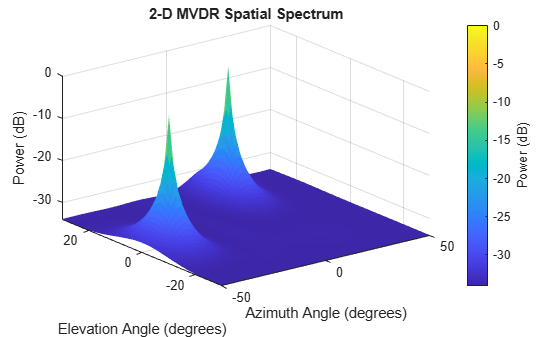# plotSpectrum

System object: phased.MVDREstimator2D
Package: phased

Plot spatial spectrum

## Syntax

```plotSpectrum(estimator) plotSpectrum(estimator,Name,Value) hl = plotSpectrum(___) ```

## Description

`plotSpectrum(estimator)` plots the spatial spectrum resulting from the most recent execution of the object.

`plotSpectrum(estimator,Name,Value)` plots the spatial spectrum with additional options specified by one or more `Name,Value` pair arguments.

`hl = plotSpectrum(___)` returns the line handle in the figure.

## Input Arguments

 `H` Spatial spectrum estimator object.

### Name-Value Arguments

Specify optional pairs of arguments as `Name1=Value1,...,NameN=ValueN`, where `Name` is the argument name and `Value` is the corresponding value. Name-value arguments must appear after other arguments, but the order of the pairs does not matter.

Before R2021a, use commas to separate each name and value, and enclose `Name` in quotes.

 `NormalizeResponse` Set this value to `true` to plot the normalized spectrum. Setting this value to `false` plots the spectrum without normalization. Default: `false` `Title` Character vector to use as figure title. Default: `''` `Unit` Plot units, specified as `'db'`, `'mag'`, or `'pow'`. Default: `'db'`

## Examples

expand all

Estimate the DOAs of two signals received by a 50-element URA with a rectangular lattice. The antenna operating frequency is 150 MHz. The actual direction of the first signal is -37° in azimuth and 0° in elevation. The direction of the second signal is 17° in azimuth and 20° in elevation.

Create signals sampled at 8 kHz.

```fc = 150e6; fs = 8000; t = (0:1/fs:1).'; x1 = cos(2*pi*t*300); x2 = cos(2*pi*t*400); array = phased.URA('Size',[5 10],'ElementSpacing',[1 0.6]); array.Element.FrequencyRange = [100e6 300e6]; x = collectPlaneWave(array,[x1 x2],[-37 0;17 20]',fc);```

`noise = 0.1*(randn(size(x))+1i*randn(size(x)));`

Create the MVDR DOA estimator for URA.

```estimator = phased.MVDREstimator2D('SensorArray',array,... 'OperatingFrequency',fc,... 'DOAOutputPort',true,'NumSignals',2,... 'AzimuthScanAngles',-50:50,... 'ElevationScanAngles',-30:30);```

Use the `step` method to the DOA estimates.

`[~,doas] = estimator(x + noise)`
```doas = 2×2 17 -37 20 0 ```

Plot the spectrum.

`plotSpectrum(estimator)`